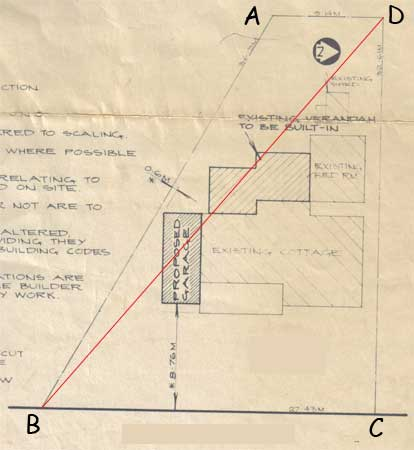My Name is Ronald. I have a building block of land with four unequal sides and only one right angle. I want to know the total area (in metres) and how the calculations were carried out. The four sides are: Rear of property: 9.14 metres left side: 36.9 metres Right side: 32.61 Metres front to street: 27.43 Metres The front to street and right side constitute a right angle. but there are no others. I am attaching a plan that shows the area and shape that might serve to assist in the calculations. Regards, Ronald Ronald, Below is part of the plan you sent. I added one line and labeled the corners of the lot.Since the angle at C is a right angle the length of CD is the height of triangle DBC and hence you can find its area using the standard expression area of DBC = 1/2 baseheight = 1/2 27.4332.61 = 447.25 square metres Again since the angle at C is a right angle I can find the length of DB using Pythagoras theorem. |DB|2 = |BC|2 + |CD|2 = 27.432 + 32.612 = 1815.81 and hence |DB| = √1815.81 = 42.61 metres. Finally since I now know the lengths of the sides of triangle ABD I can find its area using Heron's Formula. a = 42.61 metres, b = 9.14 metres and c = 36.9 metres and hence s = (42.61 + 9.14 + 36.9)/2 metres and area of ABD = Sqrt[s(s-a)(s-b)(s-c)] = 140.92 square metres. Thus the area of your lot is 447.25 + 140.92 = 588.17 square metres. Penny